In the SAS/IML language, a matrix contains data of one type: numeric or character. If you want to create a SAS data set that contains mixed-type data (numeric and character), SAS/IML 15.1 provides support to write multiple matrices to a data set by using a single statement. Specifically, the CREATE FROM and APPEND FROM statements now support writing multiple matrices of any types. SAS/IML 15.1 was released as part of SAS 9.4m6.

### Write mixed-type data from SAS/IML objects

With the new enhancements to the CREATE FROM and APPEND FROM statements, you now have four ways to write mixed type data to a SAS data set:

### In SAS/IML 15.1, you can specify multiple matrices on the CREATE FROM statement. The matrices can be any type. In the following example, X matrix is a numeric matrix and C is a character matrix: ```/* read numeric and character vars in one call */ proc iml; NumerVarNames = {'N' 'N2' 'N3'}; X = { 1 2 3, 2 4 6, 3 6 9, 4 8 12}; charVarNames = {'Animal' 'Flower'}; C = {'Rat' 'Iris', 'Pig' 'Rose', 'Goat' 'Daisy', 'Duck' 'Lily'};   /* SAS/IML 15.1: write multiple matrices of any type to a SAS data sets */ AllNames = NumerVarNames || CharVarNames; create MyData from X C [colname=AllNames]; /* specify multiple matrices */ append from X C; /* repeat matrix names */ close; QUIT;   proc print data=MyData noobs; run;```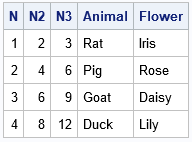Although the new enhancements to the CREATE FROM and APPEND FROM statements enable you to write mixed-type data to a SAS data set, you can also write multiple matrices regardless of the types. For example, you can use the same technique to write multiple numeric matrices. Notice that if you want to specify the names of the data set variables, you use a single COLNAME= option at the end of the CREATE FROM statement.

The post Write numeric and character matrices to a data set from SAS/IML appeared first on The DO Loop.In SAS/IML programs, a common task is to write values in a matrix to a SAS data set. For some programs, the values you want to write are in a matrix and you use the CREATE FROM/APPEND FROM syntax to create the data set, as follows:

```proc iml; X = {1 2 3, 4 5 6, 7 8 9, 10 11 12}; create MyData from X[colname={'A' 'B' 'C'}]; /* create data set and variables */ append from X; /* write all rows of X */ close; /* close the data set */```

In other programs, the results are computed inside an iterative DO loop. If you can figure out how many observations are generated inside the loop, it is smart to allocate room for the results prior to the loop, assign the rows inside the loop, and then write to a data set after the loop.

However, sometimes you do not know in advance how many results will be generated inside a loop. Examples include certain kinds of simulations and algorithms that iterate until convergence. An example is shown in the following program. Each iteration of the loop generates a different number of rows, which are appended to the Z matrix. If you do not know in advance how many rows Z will eventually contain, you cannot allocate the Z matrix prior to the loop. Instead, a common technique is to use vertical concatenation to append each new result to the previous results, as follows:

```/* sometimes it is hard to determine in advance how many rows are in the final result */ free Z; do n = 1 to 4; k = n + floor(n/2); /* number of rows */ Y = j(k , 3, n); /* k x 3 matrix */ Z = Z // Y; /* vertical concatenation of results */ end; create MyData2 from Z[colname={'A' 'B' 'C'}]; /* create data set and variables */ append from Z; /* write all rows */ close; /* close the data set */```

Concatenation within a loop tends to be inefficient. As I like to say, "friends don't let friends concatenate results inside a loop!"

If your ultimate goal is to write the observations to a data set, you can write each sub-result to the data set from inside the DO loop! The APPEND FROM statement writes whatever data are in the specified matrix, and you can call the APPEND FROM statement multiple times. Each call will write the contents of the matrix to the open data set. You can update the matrix or even change the number of rows in the matrix. For example, the following program opens the data set prior to the DO loop, appends to the data set multiple times (each time with a different number of rows), and then closes the data set after the loop ends.

```/* alternative: create data set, write to it during the loop, then close it */ Z = {. . .}; /* tell CREATE stmt that data will contain three numerical variables */ create MyData3 from Z[colname={'A' 'B' 'C'}]; /* open before the loop. The TYPE of the variables are known. */ do n = 1 to 4; k = n + floor(n/2); /* number of rows */ Z = j(k , 3, n); /* k x 3 matrix */ append from Z; /* write each block of data */ end; close; /* close the data set */```

The following output shows the contents of the MyData3 data set, which is identical to the MyData2 data set: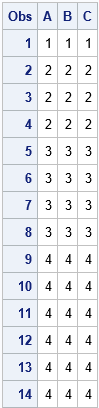Notice that the CREATE statement must know the number and type (numerical or character) of the data set variables so that it can set up the data set for writing. If you are writing character variables, you also need to specify the length of the variables. I typically use missing values to tell the CREATE statement the number and type of the variables. These values are not written to the data set. It is the APPEND statement that writes data.

I previously wrote about this technique in the article "Writing data in chunks," which was focused on writing large data set that might not fit into RAM. However, the same technique is useful for writing data when the total number of rows is not known until run time. I also use it when running simulations that generate multivariate data. This technique provides a way to write data from inside a DO loop and to avoid concatenating matrices within the loop.

The post Write to a SAS data set from inside a SAS/IML loop appeared first on The DO Loop.If you obtain data from web sites, social media, or other unstandardized data sources, you might not know the form of dates in the data. For example, the US Independence Day might be represented as "04JUL1776", "07/04/1776", "Jul 4, 1776", or "July 4, 1776." Fortunately, the ANYDTDTE informat makes it easy read dates like these into SAS.

The ANYDTDTEw. informat is a flexible alternative to older informats such as DATEw., MMDDYYw., and YYMMDDw. If your dates are in a specific form, the older informats work great and serve to document that all dates must be in that standard form. If the dates are not standardized or you need to read a string like "July 4, 1776", the ANYDTDTE informat is a godsend.

### The ANYDTDTE informat for reading dates

The following SAS DATA step shows that the ANYDTDTEw. format combines several older formats into a "super format" that attempts to convert a character string into a date. The ANYDTDTE format can not only replace many of the older formats, but it can be used to convert a string like "Jul 4, 1776" into a date, as follows:

```data Dates; input @1 Style \$8. @9 Value anydtdte12.; format Value DATE10.; datalines; DATE 04JUL1776 MMDDYY 07041776 MMDDYY 07/04/1776 YYMMDD 17760704 N/A Jul 4, 1776 N/A July 4, 1776 ;   proc print noobs; run;```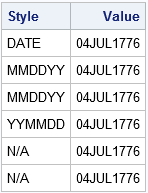As you can see, the ANYDTDTE informat reads six different strings, but converts all of them to the SAS date value that corresponds to 04JUL1776.

### MMDD or DDMM? How does ANYDTDTE interpret ambiguous dates?

The string 07/04/1776 can be interpreted as "April 7, 1776" or "July 4, 1776," depending upon the local convention. Europeans tend to interpret the string as DD/MM/YYYY whereas the US convention is to use MM/DD/YYYY. How does the ANYDTDTEw. informat guess which interpretation might be correct?

The answer is that the informat looks at the DATESTYLE SAS option. By default, the DATESTYLE option uses the LOCALE system option to guess which style to use. You can use PROC OPTIONS to see the value of these options, which are printed to the SAS log:

`proc options option=(DATESTYLE LOCALE) value; run;`
```Option Value Information For SAS Option DATESTYLE Value: MDY Scope: Default How option value set: Locale   Option Value Information For SAS Option LOCALE Value: EN_US ...```

For my system, the DATESTYLE option is set to MDY, which means that the string "07/04/1776" will be interpreted MM/DD/YYYY. If you need to read dates that obey a different convention, you can use the global OPTIONS statement to set the DATESTYLE option:

```options DATESTYLE=DMY; /* change default style convention */ /* Restore default convention: options DATESTYLE=Locale; */```

### Other "ANY" informats in SAS

There are two other SAS infomats that are similar to the ANYDTDTE informat:

Here's a tip to help you remember these seemingly cryptic names. The first part of the name is "ANYDT", which means that the input string can be ANY datetime (DT) value. The end of the name refers to the numerical value that is produced by the informat. The resulting value can be a date (DTE), a datetime (DTM), or a time (TME) value. Thus the three informats all have the mnemonic form ANYDTXXX where the XXX suffix refers to the value that is produced.

The post One informat to rule them all: Read any date into SAS appeared first on The DO Loop.In the SAS/IML language, you can read data from a SAS data set into a set of vectors (each with their own name) or into a single matrix. Beginning programmers might wonder about the advantages of each approach. When should you read data into vectors? When should you read data into a matrix?

### Read data into SAS/IML vectors

You can specify the names of data set variables in the SAS/IML READ statement, as follows:

```proc iml; use Sashelp.Class; /* open the data set */ read all var {"Name" "Height" "Weight"}; /* read 3 vars into vectors */ close Sashelp.Class; /* close the data set */```

The previous statements create three vectors, whose names are the same as the variable names. You can perform univariate analyses on the vectors, such as descriptive statistics. You can also create new variables from arbitrary transformations of the vectors, such as the following computation of the body mass index:

```BMI = weight / height##2 * 703; print BMI[rowname=Name];```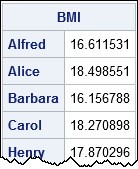Some of the advantages of reading data into vectors are:

• Variables are given informative names.
• You can use a single READ statement to read character variables and numerical variables.

When you load summarized data, you might want to read the variables into vectors. For example, to read the ParameterEstimates table from a regression analysis, you probably want to read the variable names, parameter estimates, standard errors, and p-values into separate vectors.

### Read data into a SAS/IML matrix

You can use the INTO clause in the READ statement to read data set variables into a SAS/IML matrix. All the variables have to be the same type, such as numeric. For example, the following statements read three numeric variables into a matrix:

```proc iml; varNames = {"Age" "Height" "Weight"}; /* these vars have the same type */ use Sashelp.Class; /* open the data set */ read all var varNames into X; /* read 3 vars into matrix */ close Sashelp.Class; /* close the data set */```

The matrix X contains the raw data. Each column is a variable; each row is an observation. For many descriptive statistics, you can use a single function call to compute statistics across all columns. You can also compute multivariate statistics such as a correlation matrix:

```mean = mean(X); /* mean of each column */ corr = corr(X); /* correlation matrix */ print mean[colname=varNames], corr[colname=varNames rowname=varNames];```You can use this technique to create vectors whose names are different from the names of data set variables. For example, in my blog posts I often load data into vectors named x and y to emphasize that the subsequent analysis will work for any data, not just for the example data.

Some of the advantages of reading data into a matrix are:

• You can compute descriptive statistics for all columns by using a single function call.
• You can sort, transpose, or reorder columns of the data.
• You can compute row operations, such as the sum across rows.
• You can compute multivariate statistics such as finding complete cases or computing a correlation matrix.
• Many statistical analyses, such as least squares regression, have a natural formulation in terms of matrix operations.

I usually read raw data into a matrix and summarized data into vectors, but as you can see, there are advantages to both approaches.

What technique do you use to read data into SAS/IML?

The post Read data into vectors or into a matrix: Which is better? appeared first on The DO Loop.Dear Rick,
I have a data set with 1,001 numerical variables. One variable is the response, the others are explanatory variable. How can I read the 1,000 explanatory variables into an IML matrix without typing every name?

That's a good question. You need to be able to perform two sub-tasks:

1. Create a character vector that contains the names of all the variables. (If the data set contains both numeric and character variables, the character vector should contain the names of all numeric variables.)
2. Exclude one or more elements from a character vector.

### Discover the names data set variables

Just as you can use PROC CONTENTS to discover the names of variables in a data set, SAS/IML has the CONTENTS function, which returns a character vector that contains the variable names. The argument to the CONTENTS function can be the name of a data set. If you have already opened a data set you can skip the argument to obtain the variable names of the open data set, as follows:

```proc iml; use Sashelp.Heart; /* open data set */ varNames = contents(); /* get all variable names */```

However, most of the time (as above) we do not have a data set that has only numerical variables. To obtain a vector of only the numeric variables, read one observation of the data into a matrix, and use the COLNAME= option to obtain the variable names:

```read next var _NUM_ into X[colname=varNames]; /* read only numeric vars */ print varNames;```

To save space, only the first few columns of the output are displayed below:### Exclude elements from a character vector

After you create a vector that contains variable names, you can use the SETDIF function to exclude certain variable. The SETDIF function also sorts the list of variable names, which can be useful:

```YVar = "Weight"; /* variable to exclude from the matrix */ XVarNames = setdif(varNames, YVar); /* exclude Y, sort remaining X */```

If you want to preserve the order of the variables, use the REMOVE function and specify the indices of the elements that you want to remove. The LOC function enables you to find the indices of the elements that you want to remove, as follows:

```XVarNames = remove(varNames, loc(varNames=YVar)); print XVarNames;```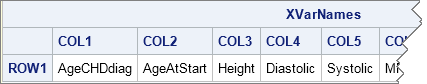### Putting it all together

For the Sashelp.Heart data set, here is how to read the variable Weight into a vector, and read all other numeric variables into a matrix X:

```proc iml; YVar = "Weight"; /* var to exclude from the matrix */ dsName = Sashelp.Heart;   use (dsName); /* open data set */ read next var _NUM_ into X[colname=varNames]; /* read only numeric vars */ XVarNames = remove(varNames, loc(varNames=YVar)); /* exclude; preserve order */   read all var YVar into Y; /* Y is vector */ read all var XVarNames into X; /* X is matrix */ close;```

You can use the LOC-ELEMENT trick to exclude multiple variables. For example, you can use the following statements to exclude two variables:

```YVar = {"Weight" "Height"}; XVarNames = remove(varNames, loc( element(varNames,YVar) ));```

The post Excluding variables: Read all but one variable into a matrix appeared first on The DO Loop.I often blog about the usefulness of vectorization in the SAS/IML language. A one-sentence summary of vectorization is "execute a small number of statements that each analyze a lot of data." In general, for matrix languages (SAS/IML, MATLAB, R, ...) vectorization is more efficient than the alternative, which is to run many statements (often inside a loop) that each analyze a small amount of data.

I usually think of vectorization as applying to computations. However, I recently realized that the same principle applies when writing data to a SAS data set. I needed to compute a large number of results and write them to a SAS data set. Because each result required a similar computation, I wrote a program that looked something like the following.

```proc iml; N = 1e5; /* 100,000 = number of observations to write */ x = j(1,5);   t0 = time(); call randseed(123); create DS1 from x[colname=("x1":"x5")]; /* open data set for writing */ do i = 1 to N; call randgen(x, "Uniform"); /* replaces complex calculation */ append from x; /* write 1 obs with 5 variables */ end; close DS1; /* close data set */   t1 = time() - t0; print t1[F=5.3]; quit;```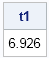In the preceding program, the call to the RANDGEN function is used in place of a complicated computation. After running the program I was disappointed. Almost 7 seconds for a mere 100,000 results?! That's terrible performance! After studying the program I began to wonder whether the problem was that I was calling the APPEND statement many times, and each call does only a little bit of work (writes one observation). That is a classic vectorize-this-step situation.

I decided to see what would happen if I accumulated 1,000 results and then output those results in a single APPEND statement. My hope was that the performance would improve by calling the APPEND statement fewer times and writing more data with each call. The rewritten program looked similar to the following:

```proc iml; N = 1e5; /* total number of observations to write */ BlockSize = 1e3; /* number of observations in each APPEND stmt */ NumIters = N/BlockSize; /* number of calls to the APPEND stmt */ x = j(1,5);   t0 = time(); call randseed(123); xChunk = j(BlockSize,5,0); create DS2 from xChunk[colname=("x1":"x5")]; do i = 1 to NumIters; do j = 1 to BlockSize; call randgen(x, "Uniform"); /* replaces complex calculation */ xChunk[j,] = x; /* accumulate results */ end; append from xChunk; /* write 1,000 results */ end; close DS2; t2 = time() - t0; print t2[F=5.3]; quit;```Ahh! That's better! By calling the APPEND statement 100 times and writing 1,000 observations for each call, the performance of the program increased dramatically.I wrote the second program so that the number of observations written by the APPEND statement (BlockSize) is a parameter. That enables me to run tests to examine the performance as a function of how many observations are written with each call. The following graph summarizes the situation.

The graph indicates that the major performance improvements occur when increasing the number of observations from 1 to 20. The curve flattens out after 50 observations. Beyond 100 observations there is no further improvement.

There are two lessons to learn from this experience:

• Writing (and reading!) data in a matrix language is similar to other numerical operations. Try to avoid reading and writing small quantities of data, such as one observation at a time. Instead, read and write larger chunks of data.
• You don't have to create huge matrices with gigabytes of data to realize the performance improvement due to vectorization. In this program, writing a matrix that has 100 rows is a vast improvement over writing one observation at a time.

The principal of vectorization tells us to execute a small number of statements that each analyze a lot of data. The same principle applies to reading and writing data in a matrix language. Avoid reading or writing one observation at a time. Instead, you can improve performance by ensuring that each I/O statement reads or writes a hundred or more observations.Many people know that the SAS/IML language enables you to read data from and write results to multiple SAS data sets. When you open a new data set, it is a good programming practice to close the previous data set. But did you know that you can have two data sets open simultaneously, one for reading and one for writing? The trick is to use the SETIN and SETOUT statements to specify the data sets that are used for reading and writing. Among other advantages, this technique enables you to use SAS/IML to analyze data that might be larger than can fit into the RAM on your computer.

This article is motivated by a question posted to the SAS/IML Support Community. The SAS/IML programmer was essentially running a BY-group analysis in SAS/IML. The user wanted to use the WHERE clause on the READ statement to read the data for each BY-group.

To illustrate the technique, consider the following BY-group regression analysis of the vehicles in the Sashelp.Cars data set. For each value of the Make variable (Acura, Audi, BMW,...), PROC REG performs an ordinary least-squares (OLS) analysis that uses the Wheelbase and Length variables to model the Weight of a vehicle. The parameter estimates are saved to the OutEst data set:

```proc reg data=sashelp.cars noprint outest=OutEst; by Make; model Weight = Wheelbase Length; run;```

Let's create a similar analysis in the SAS/IML language. Although SAS/IML is often used to perform analyses that are not available from any SAS procedure, this simple and familiar analysis will enable us to concentrate on how to read and write data sets simultaneously. The following SAS/IML statements define a module that computes the parameter estimates for an OLS model. Because some of the BY groups result in singular linear systems, the GINV function is used to solve for the parameter estimates, rather than the more efficient SOLVE function.

```proc iml; start OLSParamEst(Y, _X); X = j(nrow(_X),1,1) || _X; /* add intercept column to design matrix */ XPX = X` * X; /* cross-products */ /* handle singular systems by using GINV rather than SOLVE */ Estimate = ginv(XPX) * X`*Y; /* parameter estimates */ return( T(Estimate) ); /* return as row vector */ finish;```

The BY-group analysis will be carried out one category at a time. The following statements open the input data set and read the BY-group categories. The output data set (which will contain the parameter estimates) is also opened for writing. Notice that you need to specify the names and types of the output variables on the CREATE statement, including the length of any character variables. Lastly, the SETIN and SETOUT statements are used to specify that the data sets that will be used for subsequent reading and writing operations:

```use Sashelp.cars; /* input data */ read all var {"Make"}; /* read BY var */ byGroup = unique( Make ); /* compute unique levels */   ByVal = BlankStr(nleng(Make)); /* set length of ByVal variable (12.3) */ OutVarNames = {"Intercept" "Wheelbase" "Length"}; Estimate = {. . .}; /* output variables are numeric */ create RegOut from Estimate[r=ByVal c=OutVarNames];   setin Sashelp.Cars; /* make current for reading */ setout RegOut; /* make current for writing */```

The SETIN statement means "every time you want to read, use the Sashelp.Cars data set." The SETOUT statement means "every time you want to write, use the RegOut data set." The following statements loop over the unique categories of the Make variable, and perform an OLS analysis for the vehicles that have a common make. You can use the INDEX statement to speed up reading the BY groups.

```InVarNames = {"Weight" "Wheelbase" "Length"}; /* input variables */ index Make; /* index by BY group variable */ do i = 1 to ncol(byGroup); ByVal = byGroup[i]; read all var InVarNames where(Make=(ByVal)); /* read from Cars */ Estimate = OLSParamEst(Weight, Wheelbase||Length); append from Estimate[r=ByVal]; /* write to RegOut */ end;   close Sashelp.Cars RegOut;```

The program performs 38 parameter estimates, one for each unique value of the Make variable. Because these data will fit entirely into RAM, you could also perform this analysis by using the UNIQUE-LOC or UNIQUEBY techniques.

In summary, use the SETIN and SETOUT statements when you want to have two SAS data sets open simultaneously, one for reading and one for writing. By the way, you can also use the SETOUT statement to write to several data sets, such as writing predicted and residual values to one while writing parameter estimates to another.SAS has several kinds of special data sets whose contents are organized according to certain conventions. These special data sets are marked with the TYPE= data set attribute.

For example, the CORR procedure can create a data set with the TYPE=CORR attribute. You can decipher the structure of the data set by using the _TYPE_ variable. For example, the following PROC CORR statement creates a TYPE=CORR data set:

```/* create TYPE=CORR data set */ proc corr data=sashelp.cars out=Corr cov nomiss; var Weight Cylinders EngineSize HorsePower; quit;   proc print data=Corr noobs; run;```The covariance matrix is contained in the observations for which _TYPE_="COV". Similarly, the correlation matrix is contained in the observations for which _TYPE_="CORR". The observation for which _TYPE_="MEAN" contains the sample means, _TYPE_="STD" contains the standard deviations, and so on. Another special variable, _NAME_, identifies the rows of the covariance and correlation matrices.

How can you read a special SAS data set into matrices in the SAS/IML language? There are several ways to do this, but a useful technique is to use a WHERE clause on the READ statement to subset the observations, as follows:

```/* Read special SAS data set into SAS/IML matrices and vectors */ proc iml; use Corr; read all var _num_ where(_TYPE_="COV") into cov[r=_NAME_ c=VarNames]; read all var _num_ where(_TYPE_="CORR") into corr; read all var _num_ where(_TYPE_="MEAN") into mean; read all var _num_ where(_TYPE_="STD" ) into std; close Corr;   print cov, mean, std, corr;```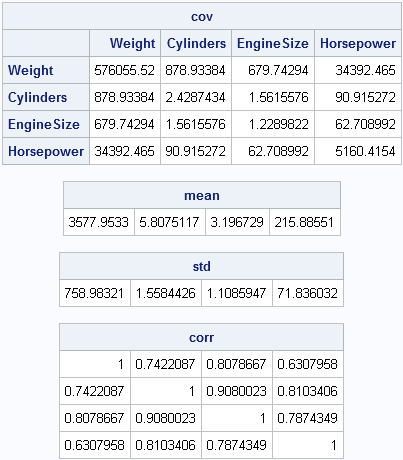Notice several points:

• The _NUM_ keyword reads all numerical variables. Therefore the same code works for any TYPE=CORR data set, not just the one in this example.
• The WHERE clause is used to select certain observations within this structured data set. Although the WHERE statements cause the data set to be read multiple times, reading the data is usually cheap compared with the computational cost of the rest of a SAS/IML program.
• The R= and C= options on the first READ statement are abbreviations for the ROWNAME= and COLNAME= options. These options do two things. First, they create two vectors, _NAME_ and VarNames, that contain the names of all numerical variables in the data set. Second, they implicitly call the MATTRIB statement to assign rown ames and column names to the cov matrix. Notice that the PRINT statement automatically printed the column and row headings for the cov matrix.

You can use this trick to read any kind of a structured data set into SAS/IML matrices. It is not always the most efficient way to read the data, but it is very general and can be used to read the "special" TYPE= data sets that are created by SAS procedures.A SAS/IML user on a discussion forum was trying to read data into a SAS/IML matrix, but the data was so large that it would not fit into memory. (Recall that SAS/IML matrices are kept in RAM.) After a few questions, it turned out that the user was trying to compute certain statistical quantities for each row of the data. This is good news because it implies that he does not have to read the entire huge data set into a SAS/IML matrix. Instead, he can read a block of observations into a matrix, do the computations for those rows, and overwrite the matrix by reading the next block. This article describes how to use the SAS/IML language to read blocks of observations from a SAS data set.

The SAS/IML language supports several ways to read data from a SAS data set. I have previously discussed sequential access and random access of data. In the article on sequential access, I showed how to read data one observation at a time, but you can also read blocks of observations.

### A simple example: Computing row means

Suppose that you have the following data, which contains 20,000 observations and 1,000 variables:

```%let NumCols = 1000; %let NumRows = 20000;   data Big; keep x1-x&NumCols; array x{&NumCols} x1-x&NumCols; call streaminit(1); do i = 1 to &NumRows; do j = 1 to &NumCols; x{j} = i; end; output; end; run;```

Each row is constant, and the mean of the ith row is i. This data set is not huge, so you do not need to do anything special to read the data. The following call to PROC IML shows the "usual" way to read the data into a SAS/IML matrix and to compute the row sums:

```proc iml; use Big nobs N; /* N = num obs in the data set */ read ALL var _NUM_ into x; close Big;   result = x[ , :]; /* compute mean of each row */ /* print the top and bottom observations */ top = 1:3; bottom = (N-3):N; print (result[top, ])[label="Result" r=(char(top))], (result[bottom, ])[r=(char(bottom))];```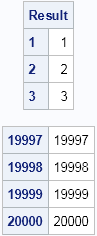The first three and last three observations are printed. Two features of the SAS/IML language are used that might not be familiar to every reader:

Obviously, if you really wanted to compute row means you could do this computation more simply by using the DATA step. This simple example is presented so that you can focus on the core issue (reading data in blocks) without being distracted by extraneous details.

### Reading blocks of observations

Now suppose that the Big data set is much, much, bigger than in this example. So big, in fact, that it cannot fit into RAM. What can you do?

Well, because this example computes quantities that depend only on rows, you can read the data in blocks (sometimes called "chunks"). The key change is to the syntax of the READ statement. Instead of using the ALL option to read all observations, use the NEXT statement and specify the number of rows that you want to read by using a macro variable. Also, you need to use the DO DATA statement to tell the SAS/IML language to keep reading data until there are no more unread observations.

For example, the following PROC IML program reads 2,000 observations at a time:

```%let BlockSize = 2000;   proc iml; use Big nobs N; /* 1. Get number of observations */   result = j(N, 1, .); /* 2. allocate space for results */ /* read the blocks */ row = 1; do data; /* 3. DO DATA statement */ read next &BlockSize var _NUM_ into x; /* 4. Read block */ lastRow = min(row + &Blocksize - 1, N); /* don't go past N */ result[row:lastRow, ] = x[ , :]; /* 5. Compute on this block */ row = lastRow + 1; end;   close Big; /* print the top and bottom observations */ top = 1:3; bottom = (N-3):N; print (result[top, ])[label="Result" r=(char(top))], (result[bottom, ])[r=(char(bottom))];```

The output is the same as before. The main features of this program are as follows:

1. As before, use the NOBS option on the USE statement to get the total number of observations.
2. Allocate space to hold the results.
3. Use the DO DATA statement to read the data.
4. Use the NEXT &BlockSize option to read 2,000 observations into the matrix x.
5. Compute and store the statistics for this block of observations.

That's it. This technique saves memory because you re-use the matrix x over and over again. You do not run out of RAM because the matrix is never huge.

In terms of efficiency, choose the block size to be fairly large. Reading the data in large blocks enables you to vectorize the computations, which is more efficient than reading and computing with small blocks.

### One more trick: Reading the last block of observations

There is one wrinkle that you might encounter when you use this technique. If the number of observations is not an integer multiple of the block size, then the last block of observations is not fully read. Instead, you get a warning the looks like the following:

```   WARNING: Only 1234 observations available, 2000 requested.
```

This is just an informational message. SAS/IML does in fact read all of the available data, and the fact that the MIN function is used to define the lastRow variable means that the number of observations read will match the index for the result matrix.

Whether this "block reading" technique is suitable for your application depends on what you are trying to accomplish. But in cases where the computations depend only on rows, you can solve your problem by reading portions of the data, even when the complete data are too large to fit into RAM.Many SAS procedures can produce ODS statistical graphics as naturally as they produce tables. Did you know that it is possible to obtain the numbers underlying an ODS statistical graph? This post shows how.

Suppose that a SAS procedure creates a graph that displays a curve and that you want the (x,y) values along the curve. Or maybe the procedure creates a scatter plot and you want the data values for each marker. Often you can scour the SAS documentation until you find some option that produces the values that you want, either in a table or in an output data set. But occasionally I've been stymied. "SAS computed the values," I've hissed through clenched teeth, "why won't it let me see them!"

Another reason to want the data in a graph is if the ODS graph isn't quite what you want. Maybe the SAS procedure creates a histogram but you prefer a box plot. Maybe you just want to make minor modifications to the graph's appearance. If you can get the data, you can use PROC SGPLOT to redraw the graph the way that you want it.

So how can you get the values that underlie an ODS statistical graph? The key observation is that every graph is an ODS "object" that has a name. Therefore you can use the ODS OUTPUT statement to write the data in the ODS object to a SAS data set.

### Getting to the data in a Q-Q Plot

As an example, suppose that you run a regression that the procedure outputs a normal quantile-quantile (Q-Q) plot of the residuals. Suppose further the you want to obtain the data used to create the plot.

You can use the ODS TRACE ON statement to find out the name of any ODS object (including a graph) that is produced by a procedure. In the following statement, PROC LOESS fits a curve to a subset of data in the Sashelp.Iris data set and creates several graphs, including a Q-Q plot with the name "QQPlot." You can use the ODS OUTPUT statement to create a SAS data set that contains the data in the Q-Q plot:

```ods graphics on; proc loess data=sashelp.iris plots=QQPlot; where Species^="Setosa"; model PetalWidth = PetalLength; ods output QQPlot = QQData; /* create data set from QQPlot */ run;```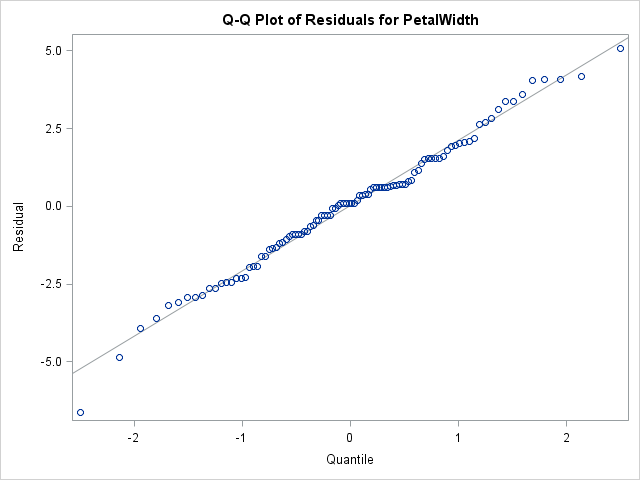The Q-Q plot is shown above. Let's see what the QQData data set looks like:

```proc contents data=QQData varnum; ods exclude Attributes EngineHost; run;```The PROC CONTENTS output shows that QQData contains six variables. Several have long names and bizarre labels. The names of the variables are generated automatically by the procedure and are not intended for "human consumption." Nevertheless, this data set contains all the data necessary to reproduce the reproduce the figure. Well, almost. The equation of the line is not in the data, but you can use PROC UNIVARIATE to find out the parameter estimates for the normal curve that best fits the residuals. The analysis is not shown, but the parameter estimates are (Mean, StdDev) = (0.02, 2.10).

### Creating a new graph of the same data

The hardest part of this process is figuring out the weird variable names. By looking at the graph and by knowing how Q-Q plots are created, you can determine the names of the X and Y variables. The following statements create a new Q-Q plot from the data that underlies the Q-Q plot of the loess residuals.

```proc sgplot data=QQData noautolegend; title "Normal Q-Q Plot of Loess Residuals"; scatter x=PROBIT___NUMERATE_SORT_DROPMISSI y=SORT_DROPMISSING_RESIDUAL__; lineparm x=0 y=0.02 slope=2.10; /* intercept, slope */ xaxis grid label="Normal Quantile"; yaxis grid label="Loess Residual"; run;```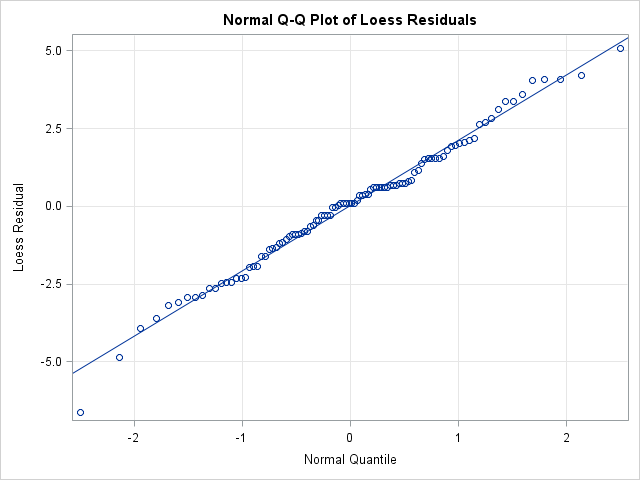The LINEPARM statement draws the diagonal reference line in the Q-Q plot. Although the data are the same, the new plot has different labels, a grid, and a descriptive title. (Another way to change the appearance is to edit the GTL template or to use the ODS Graphics Editor.)

So next time you want to get the data in an ODS graph, remember that graphs are ODS objects and that you can use the ODS OUTPUT statement to the write the data to a data set. If you can make sense of the cryptic variable names, this technique provides the values that are associated with graphical elements. You can use the values in computations or to create a modified version of the graph.